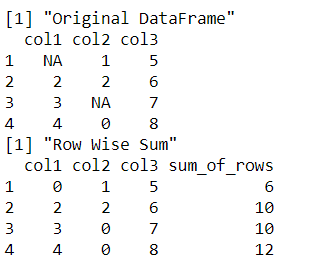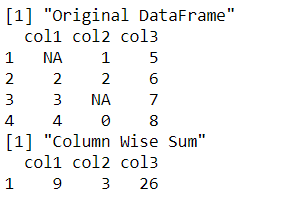# Sum Across Multiple Rows and Columns Using dplyr Package in R

• Last Updated : 14 Sep, 2021

In this article, we are going to see how to sum multiple Rows and columns using Dplyr Package in R Programming language.

The dplyr package is used to perform simulations in the data by performing manipulations and transformations. It can be installed into the working space using the following command :

`install.packages("dplyr")`

## Calculating row sums

The is.na() method in R is used to check if the variable value is equivalent to NA or not. This is important since the result of most of the arithmetic operations with NA value is NA. The replace() method in R can be used to replace the value of a variable in a data frame. This method is applied over the input data frame’s all cells and swapped with a 0 wherever found.

Syntax: replace(data, replace-val)

The mutate() method is then applied over the output data frame, to modify the structure of the data frame by modifying the structure of the data frame. New columns or rows can be added or modified in the existing data frame. A new column name can be mentioned in the method argument and assigned to a pre-defined R function.

Syntax: mutate(new-col-name = rowSums(.))

The rowSums() method is used to calculate the sum of each row and then append the value at the end of each row under the new column name specified. The argument . is used to apply the function over all the cells of the data frame.

Syntax: rowSums(.)

Code:

## R

 `library``(``"dplyr"``)`` ` `# creating a data frame``data_frame <- ``data.frame``(col1 = ``c``(``NA``,2,3,4),``                         ``col2 = ``c``(1,2,``NA``,0),``                         ``col3 = ``c``(5,6,7,8)``                         ``)``print``(``"Original DataFrame"``)``print``(data_frame)`` ` `# eliminating NA values``data_without_na <- data_frame %>%                      ``  ``replace``(``is.na``(.), 0) ``print``(``"Row Wise Sum"``)`` ` `data_mod <- data_without_na%>%``  ``mutate``(sum_of_rows = ``rowSums``(.))``print``(data_mod)`

Output:## Calculating column sums

The NA values, if present, can be removed from the data frame using the replace() method in R. Successively, the data frame is then subjected to a method summarise_all() which is applied to every variable in the data frame. It takes as argument the function sum to calculate the sum over each column of the data frame.

Syntax: summarise_all (sum)

Code:

## R

 `library``(``"dplyr"``)`` ` `# creating a data frame``data_frame <- ``data.frame``(col1 = ``c``(``NA``,2,3,4),``                         ``col2 = ``c``(1,2,``NA``,0),``                         ``col3 = ``c``(5,6,7,8)``                         ``)``print``(``"Original DataFrame"``)``print``(data_frame)`` ` `# eliminating NA values``data_without_na <- data_frame %>%                      ``  ``replace``(``is.na``(.), 0) ``print``(``"Column Wise Sum"``)`` ` `# computing column wise sum``data_mod <- data_without_na%>%``  ``summarise_all``(sum)`` ` `# printing final output``print``(data_mod)`

Output:My Personal Notes arrow_drop_up